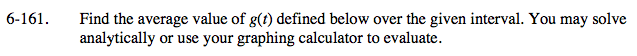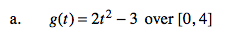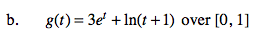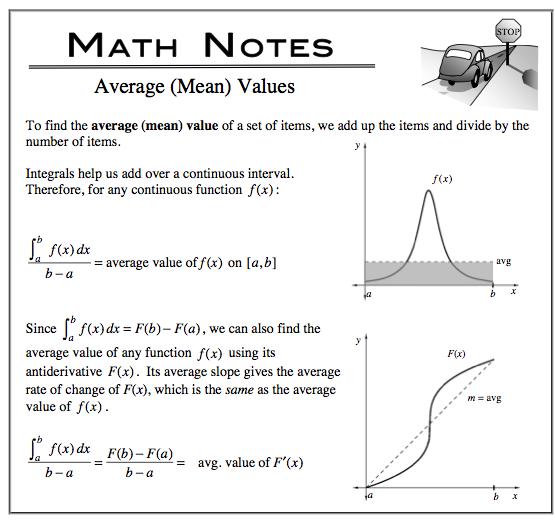### Home > CALC > Chapter 6 > Lesson 6.4.3 > Problem6-161

6-161.Examine the Math Note about about Mean (average) Value. Notice that there are two strategies to compute Mean (average) Value.

Strategy 1: compute the average area under the curve.
Strategy 2: compute a slope of a secant line.

WARNING: These strategies can NOT be used interchangeably. The strategy you choose depends on the function you are given:
f(x) or F(x).

And it depends on the question you are being asked:
Find average y-value? or Find average rate of change?

In problem 6-161, you are asked to find the average y-value of the given function g(x).